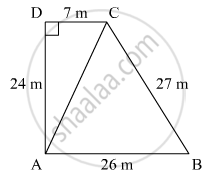# Area of Pqrs = Area of Pqr + Area of δPqs = (6+9.166)𝑐𝑚2=15.166𝑐𝑚2 - Mathematics

Short Note

Area of PQRS = Area of PQR + Area of ΔPQS = (6+9.166)𝑐𝑚2=15.166𝑐𝑚2

The sides of a quadrangular field, taken in order are 26 m, 27 m, 7 m are 24 m respectively. The angle contained by the last two sides is a right angle. Find its area.

#### Solution

The sides of a quadrilateral field taken order as AB = 26mBC = 27 m
CD = 7m and DA = 24 m
Diagonal AC is joined
By applying Pythagoras theorem

⇒AC^2=AD^2+CD^2

⇒AC=sqrt(AD^2 +CD^2)

⇒AC=sqrt(24^2+7^2)

⇒AC=sqrt625=25 m

Now area of ΔABC

S=1/2(AB+BC+CA)=1/2(26+27+25)=78/2=39 m

By using heron’s formula

Area (ΔABC) = sqrt(S(S-AD)(S_BC)(S-CA))

=sqrt(39(39-26)(39-21)(39-25))

sqrt(39xx14xx13xx12xx1)

=291.849cm^2

S=1/2(AD+CD+AC)

=1/2(25+24+7)=28cm

By using heron’s formula

∴ Area   of   ΔADC = sqrt(S(S-AD)(S-DC)(S-CA))

=sqrt(28(28-24)(28-7)(28-25))

=84m^2

∴ Area of rectangular field ABCD = area of ΔABC + area of ΔADC
= 291.849+84
= 375.8m^2

Concept: Application of Heron’s Formula in Finding Areas of Quadrilaterals
Is there an error in this question or solution?

#### APPEARS IN

RD Sharma Mathematics for Class 9
Chapter 17 Heron’s Formula
Exercise 17.2 | Q 2 | Page 19

Share